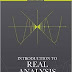In mathematics, real analysis is the theory of real numbers and real functions, which are real-valued functions of a real variable. Real analysis studies properties of real functions like convergence, limits, continuity, smoothness, differentiability and integrability. Real analysis and complex analysis are both branches of mathematical analysis.

The theorems of real analysis rely intimately upon the structure of the real number line. The real number system consists of a set ( R ), together with two binary operations denoted + and ⋅, and an order denoted <. The operations make the real numbers a field, and, along with the order an ordered field. The real number system is the unique complete ordered field, in the sense that any other complete ordered field is isomorphic to it. Intuitively, completeness means that there are no 'gaps' in the real numbers. In particular, this property distinguishes the real numbers from other ordered fields (e.g., the rational numbers Q ) and is critical to the proof of several key properties of functions of the real numbers. The completeness of the reals is often conveniently expressed as the least upper bound property.

LOOK AT: does now not personal this e-book neither created nor scanned. We simply imparting the hyperlink already available on net. If any manner it violates the law or has any issues then kindly mail us: [email protected] or contact Us for this(link removal)..

REAL ANALYSIS:-TOPOLOGY ON R AND R HAND WRITTEN NOTEReviewed by Gaurav Rathore on August 26, 2018 Rating: 5